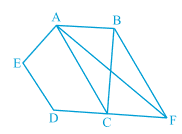Q

# In Fig. 9.27, ABCDE is a pentagon. A line through B parallel to AC meets DC produced at F. Show that (i) ar (ACB) = ar (ACF) (ii) ar (AEDF) = ar (ABCDE)

Q : 11     In Fig. , ABCDE is a pentagon. A line through B parallel to AC meets DC produced at F. Show that

(i)
(ii)ViewsWe have a pentagon ABCDE in which BF || AC and CD  is produced to F.

(i) Since ACB and ACF are on the same base AC and between same parallels AC and FB.
ar(ACB) = ar (ACF)..................(i)

(ii) Adding the ar (AEDC) on both sides in  equation (i),  we get

ar(ACB) + ar(AEDC) =  ar (ACF) + ar(AEDC)

Hence proved.

Exams
Articles
Questions